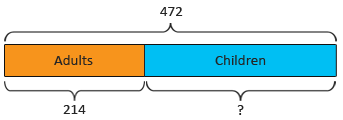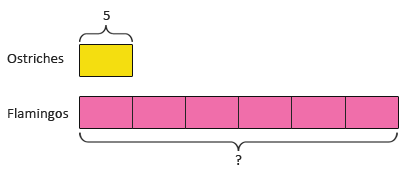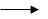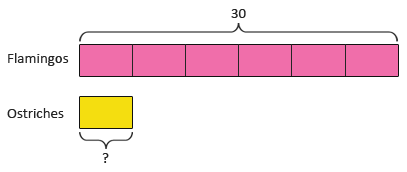# What is Singapore Math?

Singapore Maths is a way of learning and mastering essential mathematical skills and concepts at the elementary (primary) and middle school (secondary) levels with a strong emphasis on problem solving.

A distinguishing feature of the Singapore primary mathematics curriculum is the use of the Model Method for problem solving. The Model Method or Bar Model Method, in use since the 1980s, is a child-friendly pictorial language used to develop students' understanding of fundamental mathematics concepts and proficiency in solving basic math word problems.

Historically, students have always had difficulty with problem sums in the early years of primary school. To address this issue, the Ministry of Education (MOE), the central education authority in Singapore, advocated the Concrete-Pictorial-Abstract approach. This approach makes use of everyday experiences, learning aids and pictorial representations to help students understand abstract concepts.

Here are a few simple examples to illustrate the use of the heuristic model method for problem solving:
214 adults and 258 children visited Singapore Zoo last Thursday. How many people visited the zoo last Thursday?214 + 258 = 472
472 people visited the zoo last Thursday.
The bar is made of two parts which collectively make a whole. By adding the two parts (the number of adults i.e. 214, and the number of children i.e. 258), students can find the whole (the total number of people, shown by the symbol ?).
472 people visited Singapore Zoo last Thursday. If there were 214 adults, how many children were there?472 − 214 = 258
There were 258 children.
Here the whole (the total number of people) and one part (the number of adults) are given. The other part (the number of children, shown by the symbol ?) can be found by subtracting the given part (214) from the whole (472).
There are 5 ostriches at Singapore Zoo. There are 6 times as many flamingos as ostriches at the zoo. How many flamingos are there at the zoo?1 unit5 6 units6 × 5 = 30
There are 30 flamingos at the zoo.
The model above is made of two bars of lengths 6 units (the number of flamingos) and 1 unit (the number of ostriches) to show a comparison between the two quantities. The model shows that the number of flamingos is 6 times the number of ostriches. Given the number of ostriches (1 unit = 5), we can find the number of flamingos (6 units) by multiplication.
There are 30 flamingos at Singapore Zoo. There are 6 times as many flamingos as ostriches at the zoo. How many ostriches are there at the zoo?6 units30 1 unit30 ÷ 6 = 5
There are 5 ostriches at the zoo.
Given the number of flamingos (6 units), we can find the number of ostriches (1 unit) by division.

# Singapore Maths at Home Campus

Singapore school children have consistently been among the top since the 1990s in the Trends in International Mathematics and Science Study (TIMSS), an international assessment of the mathematics and science knowledge of 4th and 8th graders around the world.
The way the subject is taught in Singapore provides a strong foundation for the learning of mathematics from the primary to secondary levels and beyond.

At Home Campus, we believe that everybody can be good at mathematics, as has been proved in Singapore. As such, we follow the latest curriculum set by MOE in all the modules at Home Campus - Learn, Practice and Worksheets.

Videos and notes in the Learn module of Home Campus extensively use pictorial models like bar models, number bonds, place value charts, etc. to explain each concept. Explanations are mostly just a few words in highlighted boxes making it easy to read and understand the concept.

The Practice and Worksheets modules heavily use everyday real-life examples in problems with solutions explained either pictorially or in a few easy-to-follow steps.

These visuals provide students with a simple yet powerful tool to represent quantities and their relationships given in a problem and then work out strategies to solve the problem. Students learn when to add, subtract, multiply or divide, relying on the meaning of the problem situation rather than relying on clue-words or memorizing rules. Models are also a useful aid to help students understand the concepts of fractions, percentages and ratio.

The various tools at Home Campus allow students to master basic math skills at a more rapid pace. Students begin solving simple multi-step word problems in third grade, using models. Later grades apply this same method to more and more difficult problems. By the end of the sixth grade, students have mastered complex topics and are comfortable doing difficult multi-step word problems that are traditionally solved using Algebra.

As the Singapore maths curriculum becomes popular, more and more educators worldwide recognize its benefits. Home Campus is here to support students and educators adopt the Singapore way of learning and teaching math.